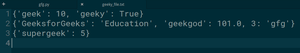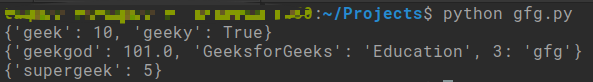GeeksforGeeks App
Open AppBrowser
Continue

# Read List of Dictionaries from File in Python

A dictionary in Python is a collection where every value is mapped to a key. Since they are unordered and there is no constraint on the data type of values and keys stored in the dictionary, it is tricky to read dictionaries from files in Python.

### Approach

The problem statement mentions a list of dictionaries that have to be read from a file. There can be two ways of doing this:

We can read data from a text file as a string and convert that data into a dictionary in Python. Assuming our data is stored in a text file in the following format –

```{'geek': 10, 'geeky': True}
{'GeeksforGeeks': 'Education', 'geekgod': 101.0, 3: 'gfg'}
{'supergeek': 5}```

The approach would include the following steps:

• Open the text file in read mode and read the content
• Parse every line in the content into a dictionary.

The pickle module in Python is mostly used in fields like Data Science where data persistence is critical. The pickle module stores the given data as a serialized byte sequence into files which can be easily retrieved at a later time. Pickle module supports various Python objects and dictionaries are one among them. This method would include the following steps:

• Importing the pickle module
• Opening the file in read binary mode
• Loading the data into a variable using pickle module’s dump method
`list_dictionary = pickle.load(filehandler)`

Below are the examples of the above-discussed approaches.

Input File:## Python3

 `def` `parse(d):``    ``dictionary ``=` `dict``()``    ``# Removes curly braces and splits the pairs into a list``    ``pairs ``=` `d.strip(``'{}'``).split(``', '``)``    ``for` `i ``in` `pairs:``        ``pair ``=` `i.split(``': '``)``        ``# Other symbols from the key-value pair should be stripped.``        ``dictionary[pair[``0``].strip(``'\'\'\"\"'``)] ``=` `pair[``1``].strip(``'\'\'\"\"'``)``    ``return` `dictionary``try``:``    ``geeky_file ``=` `open``(``'geeky_file.txt'``, ``'rt'``)``    ``lines ``=` `geeky_file.read().split(``'\n'``)``    ``for` `l ``in` `lines:``        ``if` `l !``=` `'':``            ``dictionary ``=` `parse(l)``            ``print``(dictionary)``    ``geeky_file.close()``except``:``    ``print``(``"Something unexpected occurred!"``)`

Output:

```{'geek': '10', 'geeky': 'True'}
{'GeeksforGeeks': 'Education', 'geekgod': '101.0', '3': 'gfg'}
{'supergeek': '5'}```

Time complexity: O(n*m), where n is the number of lines in the file and m is the number of key-value pairs in each line.

Auxiliary space: O(m), where m is the maximum number of key-value pairs in a single line.

 `import` `pickle`  `try``:``    ``geeky_file ``=` `open``(``'GFG.txt'``, ``'r'``)``    ``dictionary_list ``=` `pickle.load(geeky_file)``    ` `    ``for` `d ``in` `dictionary_list:``        ``print``(d)``    ``geeky_file.close()` `except``:``    ``print``(``"Something unexpected occurred!"``)`